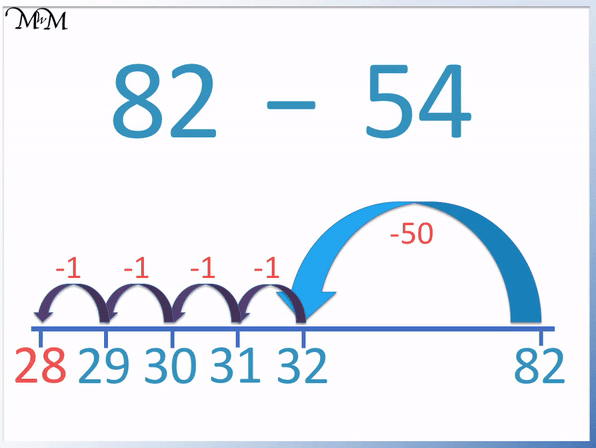# All About the Bridging to 10 Strategy

All About the Bridging to 10 StrategyThe bridging to 10 strategy is a method used to add two numbers. First add part of the number to get to 10 and then add the remainder.• Here we will add 6 to 9.
• 6 is equal to 1 plus 5.
• We add 1 to 9 to get to 10.
• We then add the remainder of 5 to 10 to get to 15.
• We calculated 9 + 1 = 10 and then 10 + 5 = 15.
• In total we have added 1 and 5, which is the same as adding 6.
• The bridging to 10 strategy is useful because it makes addition easier.
• This is because it uses number bonds to 10, which are commonly memorised.
• It is also easy to add a number to 10.• We add 18 + 8 using the bridging to 10 strategy.
• The next ten after 18 is 20.
• We first add 2 to 18 to make 20.
• We have added 2 and we need to add 6 more in order to add 8 in total.
• We add 6 more to 20 to make 26.
• 18 + 8 = 26.Supporting Lessons

#### Bridging to 10 Video Lesson# Bridging to 10

## What is Bridging to 10?

Bridging to 10 is a method of adding two numbers that have an answer larger than 10. Count up to 10 and then add on the remainder. For example, to work out 7 + 4, firstly do 7 + 3 = 10 and then add the remainder of 1 to make 11.The bridging to 10 strategy is often referred to as the bridging through 10 strategy or simply the bridging 10 strategy.

The bridging 10 strategy is a useful strategy for mental addition.

## How to Teach Bridging to 10

When learning the bridging to 10 strategy, it is first necessary to have a strong understanding of the number bonds to 10. Number lines help to teach bridging to 10 because they can be used to count up to the next ten. Furthermore, tens frames and counters can be used to help count up to the next ten.

For example, the number line below shows the bridging to 10 of the addition 7 + 5.

We can count on by 3 to reach ten and then see that 2 more must be added to add 5 in total.It helps to know the number bonds to 10 very well before learning the bridging to 10 method. This way we know that 3 must be added to 7 to make 10.

Tens frames can be used to help teach the idea of number bonds to ten and they can also be used to teach the bridging to 10 process.Tens frames allow us to easily see how many more must be added to a number in order to make ten. This helps us with the first step of the bridging ten process.

## Addition by Bridging to 10

To add two numbers by bridging to 10:

1. Find the number that when added to the larger of the two numbers makes the next multiple of ten.
2. Find the remainder by subtracting this same number from the smaller number.
3. Add this remainder onto the multiple of ten found in step 1.

For example, add 46 + 7 by bridging to 10.Step 1. Find the number that when added to the larger of the two numbers makes the next multiple of ten

46 is the larger of the two numbers. We can add 4 to 46 to make the next multiple of ten which is 50.

Step 2. Find the remainder by subtracting this same number from the smaller number

We subtract 4 from 7 to make 3. This means that the remainder is 3.

Step 3. Add this remainder onto the multiple of ten found in step 1

The remainder is 3. We add this to the multiple of ten found in step 1, which was 50.

50 + 3 = 53.

Therefore 46 + 7 = 53.

We bridged to the next ten along which was 50. Since we added 4, we needed to add 3 more to add a total of 7.

## Subtraction by Bridging to 10

To subtract by bridging to 10:

1. Subtract the ones digit of the larger number from the larger number to make a multiple of ten.
2. Find how much more must be subtracted in order to subtract the amount required.
3. Subtract this extra amount from the multiple of ten to obtain the answer to the subtraction.

For example, calculate 42 – 7 using the bridging to ten subtraction strategy.Step 1. Subtract the ones digit of the larger number from the larger number to make a multiple of ten

We subtract 2 from 42 to obtain 40.

Step 2. Find how much more must be subtracted in order to subtract the amount required

We need to subtract 7 and we have already subtracted 2.

We need to subtract 5 more because 2 + 5 = 7.

Step 3. Subtract this extra amount from the multiple of ten to obtain the answer to the subtraction

We subtract 5 more from 40 to make 35.

Therefore 42 – 7 = 35.

We subtracted 2 to bridge ten at 40 and then subtracted 5 more to subtract a total of 7.Now try our lesson on Subtraction on an Empty Number Line where we learn how to subtract a large number in chunks.error: Content is protected !!